# Pure Python library for reading DHT sensor on Pycom boards

• @Jurassic-Pork Just one small change:
The initial high period of 25 ms is too short. That had the effect that the first call failed, and it worked only from the second call on. I had to extend that to 500 ms. You can also set the Pin high in init() and skip the call.

why 500ms ? the only specification that i have seen for this is :
When power is supplied to sensor, don't send any instruction to the sensor within one second to pass unstable status.

For this a time.sleep of one second in the constructor of the class (init) ?
and :
Is the time.sleep of one second at the beginning of the read method is still necessary ?

specification for the start signal of the AM2302 sensor : min : 0.8 ms typical : 1 ms max : 20 ms
specification for the start signal of the DTH11 sensor : must be > 18 ms
So i will change the start signal value with a value of 19 ms which does the job for the two sensors.

• Great! Now working on the Lopy.
Thanks a lot @Jurassic-Pork & @robert-hh!

``````PYB: soft reboot
==========Starting main.py==========

<DTHResult object at 3ffe2ed0> <class 'DTHResult'>
True
Temperature: 21.70
Humidity: 57.50
``````

• @livius The shortest event to be captured is represented by 3 times calling __pin(). What you suggest is in addition to the pin read and loop maintenance adding the load/inc/store operation, not to mention the bookkeeping for the index I between the loops. It still could be feasible, so it's to be tested, and not to mention the additional code required to avoid a lock-up situation.

• @Jurassic-Pork
I am realy do not understand why all do this in this way

``````for i in range(len(m)):
m[i] = self.__pin()
``````

i know that time is critical
but why not do this with really shorter buffer
I know that time is critical here but `inc` should work fast
this is not whole algorithm but:

``````while  self.__pin():
m[i]+=1
while not self.__pin():
m[i]-=1
``````

and result buffer will be e.g.

``````8, -7, 14, ...
``````

• @robert-hh
ok , thanks robert .
i have made this changes in the dht.py :
1 - in the init :
def init(self, pin, sensor=0):
self.__pin = pin
self.__dhttype = sensor
self.__pin(1)
time.sleep(0.5)

and also comment this in the read method :
#self.__send_and_sleep(1, 0.025)
2 - in the collect_input method :
m = bytearray(800)

bytearray(800) ---> 857 bytes
*800 --> 6464 bytes

With my SiPy and my dth11 sensor no error in a loop of 100 measures.

• @Jurassic-Pork Just one small change:
The initial high period of 25 ms is too short. That had the effect that the first call failed, and it worked only from the second call on. I had to extend that to 500 ms. You can also set the Pin high in init() and skip the call.
I also simplified __collect_input(), saving quite some memory.

``````    def __collect_input(self):
m = bytearray(800)        # needs long sample size to grab all the bits from the DHT
irqf = disable_irq()
self.__pin(1)
for i in range(len(m)):
m[i] = self.__pin()      ## sample input and store value
enable_irq(irqf)
return m
``````

I do not think that the LoPy is faster. I assume that the sensor I issues a different collections of 1s and 0s, either caused by the different coding or the environmental conditions.

• @robert-hh
i have made the changes : 20 ms for low pulse and a buffer of 800 bits. It always works for my pycom SiPy and my DTH11 sensor. Can you tell me if it is OK for you. The changes are on my Github projet (file dth.py).

• @robert-hh ²
hello robert,
thanks for your comments. I will change my code with your comments. its seems that your pycom is faster than mine so i will change the size of the buffer.

• @Jurassic-Pork I fully understand you code. And I had enabled the print statement in you code. But with the setting you use, the device is simply not responding. Reason: the initial low code is too long. You set it to 40 ms, but it ought to be 20 ms. See here the response to a 20 ms pulse: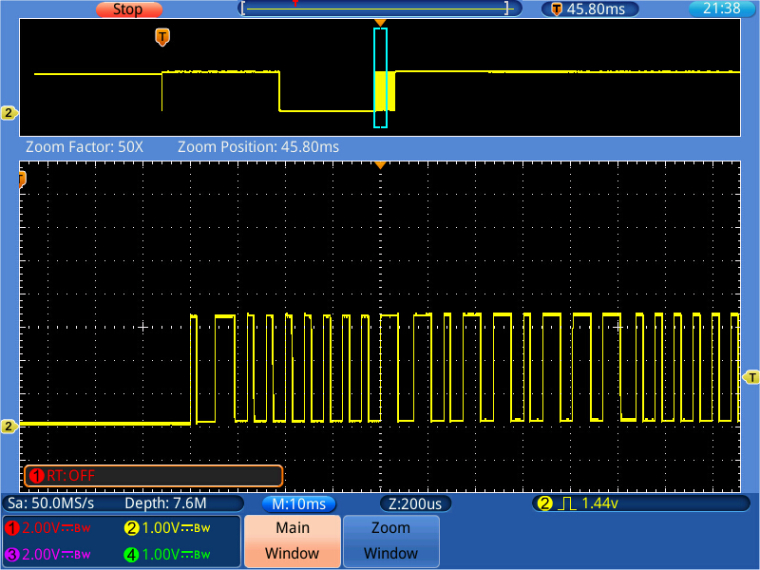You can clearly see the burst of data after the end of the pulse. Compared to that the 40 ms pulse, you've chosen: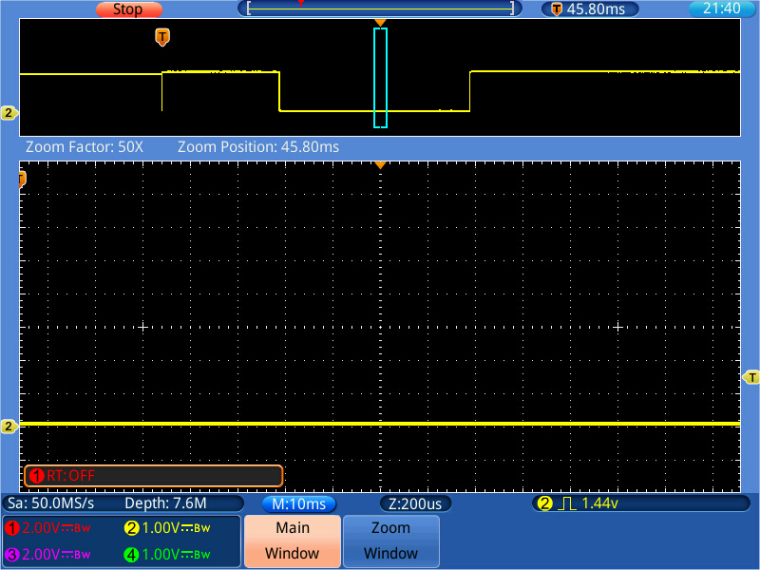No data.
The second change I had to make was increasing the raw data buffer m[], which simply was too short. I increased the size from 300 to 500. In a wort case, this would not even be enough, becase we have 40 high/low cycles. The low have a length of 10, and the hig cycles a length of 3 or 10. So in the worst case we'd need a buffer length of 800, but 500 works so far.

• @RobTuDelft Here is the code of dth.py which works for me. It still contains the print statements for some intermediate data.

``````import time
from machine import enable_irq, disable_irq,  Pin

class DTHResult:
'DHT sensor result returned by DHT.read() method'

ERR_NO_ERROR = 0
ERR_MISSING_DATA = 1
ERR_CRC = 2

error_code = ERR_NO_ERROR
temperature = -1
humidity = -1

def __init__(self, error_code, temperature, humidity):
self.error_code = error_code
self.temperature = temperature
self.humidity = humidity

def is_valid(self):
return self.error_code == DTHResult.ERR_NO_ERROR

class DTH:
'DHT sensor (dht11, dht21,dht22) reader class for Pycom'

__pin = Pin('P3', mode=Pin.OPEN_DRAIN)
__dhttype = 0

def __init__(self, pin, sensor=0):
self.__pin = pin
self.__pin(1)
self.__dhttype = sensor

time.sleep(1)

# send initial high
#self.__send_and_sleep(1, 25000)

# pull down to low
#self.__send_and_sleep(0, 40000)

# collect data into an array
data = self.__collect_input()

# parse lengths of all data pull up periods
pull_up_lengths = self.__parse_data_pull_up_lengths(data)
# if bit count mismatch, return error (4 byte data + 1 byte checksum)
print(pull_up_lengths)
if len(pull_up_lengths) != 40:
return DTHResult(DTHResult.ERR_MISSING_DATA, 0, 0)

# calculate bits from lengths of the pull up periods
bits = self.__calculate_bits(pull_up_lengths)

# we have the bits, calculate bytes
the_bytes = self.__bits_to_bytes(bits)
print(the_bytes)
# calculate checksum and check
checksum = self.__calculate_checksum(the_bytes)
if the_bytes != checksum:
return DTHResult(DTHResult.ERR_CRC, 0, 0)

# ok, we have valid data, return it
[int_rh, dec_rh, int_t, dec_t, csum] = the_bytes
if self.__dhttype==0:		#dht11
rh = int_rh		#dht11 20% ~ 90%
t = int_t	#dht11 0..50°C
else:			#dht21,dht22
rh = ((int_rh * 256) + dec_rh)/10
t = (((int_t & 0x7F) * 256) + dec_t)/10
if (int_t & 0x80) > 0:
t *= -1
return DTHResult(DTHResult.ERR_NO_ERROR, t, rh)

def __send_and_sleep(self, output, mysleep):
self.__pin(output)
time.sleep_us(mysleep)

def __collect_input(self):
# collect the data while unchanged found
unchanged_count = 0
# this is used to determine where is the end of the data
max_unchanged_count = 100
last = -1
data = []
m = *500	   # needs long sample size to grab all the bits from the DHT
self.__pin(0)
time.sleep_us(20000)
self.__pin(1)
irqf = disable_irq()
for i in range(len(m)):
m[i] = self.__pin()      ## sample input and store value
enable_irq(irqf)
for i in range(len(m)):
current = m[i]
data.append(current)
if last != current:
unchanged_count = 0
last = current
else:
unchanged_count += 1
if unchanged_count > max_unchanged_count:
break
#print(data)
return data

def __parse_data_pull_up_lengths(self, data):
STATE_INIT_PULL_DOWN = 1
STATE_INIT_PULL_UP = 2
STATE_DATA_FIRST_PULL_DOWN = 3
STATE_DATA_PULL_UP = 4
STATE_DATA_PULL_DOWN = 5

state = STATE_INIT_PULL_UP

lengths = [] # will contain the lengths of data pull up periods
current_length = 0 # will contain the length of the previous period

for i in range(len(data)):

current = data[i]
current_length += 1

if state == STATE_INIT_PULL_DOWN:
if current == 0:
# ok, we got the initial pull down
state = STATE_INIT_PULL_UP
continue
else:
continue
if state == STATE_INIT_PULL_UP:
if current == 1:
# ok, we got the initial pull up
state = STATE_DATA_FIRST_PULL_DOWN
continue
else:
continue
if state == STATE_DATA_FIRST_PULL_DOWN:
if current == 0:
# we have the initial pull down, the next will be the data pull up
state = STATE_DATA_PULL_UP
continue
else:
continue
if state == STATE_DATA_PULL_UP:
if current == 1:
# data pulled up, the length of this pull up will determine whether it is 0 or 1
current_length = 0
state = STATE_DATA_PULL_DOWN
continue
else:
continue
if state == STATE_DATA_PULL_DOWN:
if current == 0:
# pulled down, we store the length of the previous pull up period
lengths.append(current_length)
state = STATE_DATA_PULL_UP
continue
else:
continue

return lengths

def __calculate_bits(self, pull_up_lengths):
# find shortest and longest period
shortest_pull_up = 1000
longest_pull_up = 0

for i in range(0, len(pull_up_lengths)):
length = pull_up_lengths[i]
if length < shortest_pull_up:
shortest_pull_up = length
if length > longest_pull_up:
longest_pull_up = length

# use the halfway to determine whether the period it is long or short
halfway = shortest_pull_up + (longest_pull_up - shortest_pull_up) / 2
bits = []

for i in range(0, len(pull_up_lengths)):
bit = False
if pull_up_lengths[i] > halfway:
bit = True
bits.append(bit)

return bits

def __bits_to_bytes(self, bits):
the_bytes = []
byte = 0

for i in range(0, len(bits)):
byte = byte << 1
if (bits[i]):
byte = byte | 1
else:
byte = byte | 0
if ((i + 1) % 8 == 0):
the_bytes.append(byte)
byte = 0
#print(the_bytes)
return the_bytes

def __calculate_checksum(self, the_bytes):
return the_bytes + the_bytes + the_bytes + the_bytes & 255
``````

• @robert-hh
can you add the lines 46, 51, 52 in the code of method read in the file dth.py to see what is wrong :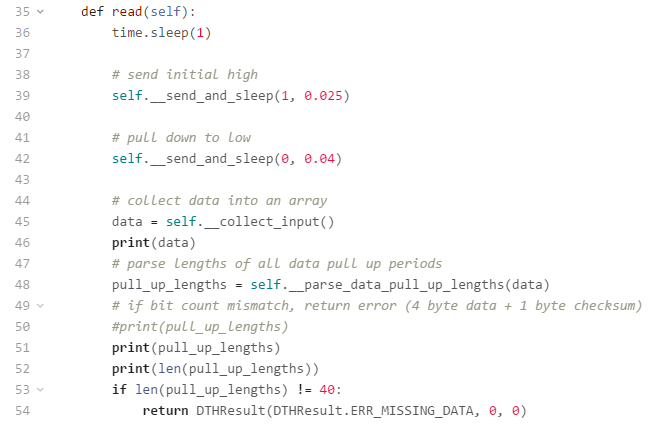you must see something like this :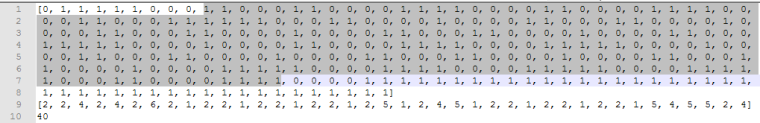i know that timing is crucial to record the data.
Timing of the AM2302 :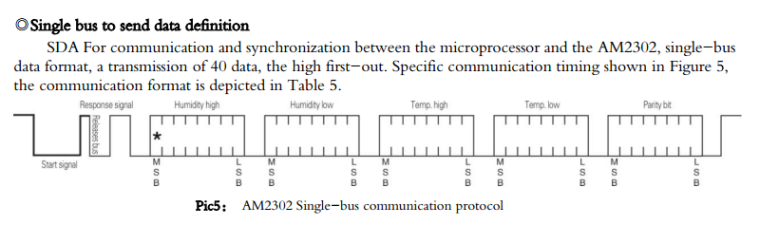The start signal is generated in the source code of dth.py by :
# pull down to low
self.__send_and_sleep(0, 0.04)

The parse_data_pull_up_lengths method is used to skip the sequence after the start signal (response signal) and to "capture" only the 40 bits of data.

• @robert-hh Could you maybe post a full code example where the DHT22 works on the Lopy?

• @Jurassic-Pork No result with a DHT22 & LoPy. The same sensor works fine with the code, found below in the thread you referred to https://forum.pycom.io/topic/948/dht-11-sensor-always-return-same-value/14. I could make it run by:

a) set the output pin to 1 in the init method.
b) removed the two calls to __send_and_sleep() in method read()
c) Increased the buffer size to 500
d) changed the start of __collect_input to:

``````    def __collect_input(self):
# collect the data while unchanged found
unchanged_count = 0
# this is used to determine where is the end of the data
max_unchanged_count = 100
last = -1
data = []
m = *500	   # needs long sample size to grab all the bits from the DHT
self.__pin(0)
time.sleep_us(20000)
self.__pin(1)
irqf = disable_irq()
for i in range(len(m)):
m[i] = self.__pin()      ## sample input and store value
enable_irq(irqf)
for i in range(len(m)):
current = m[i]
data.append(current)
if last != current:
unchanged_count = 0
last = current
else:
unchanged_count += 1
if unchanged_count > max_unchanged_count:
break
#print(data)
return data
``````

It is essential, at least for the sensor model I use, that the starting low pulse is immediately before the reading sequence and not too long.

• @RobTuDelft
i use a grove module with a DTH11 sensor ( blue ) and a pull up resistor.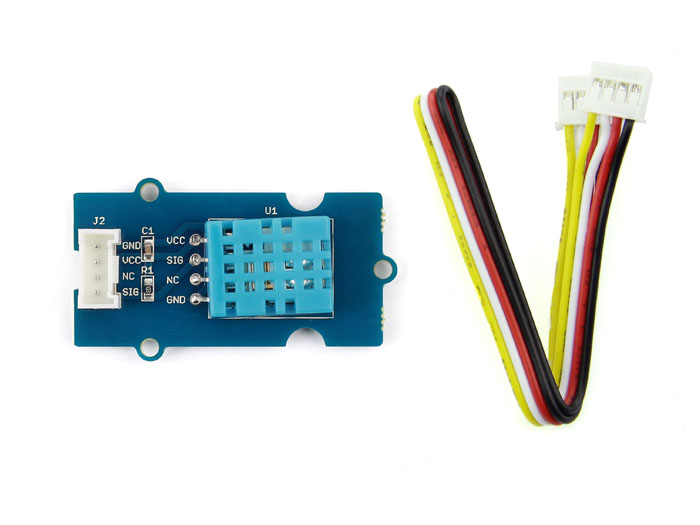What is the value of :
possible values :
ERR_NO_ERROR = 0
ERR_MISSING_DATA = 1
ERR_CRC = 2

• @RobTuDelft
Do you have pullup resistor on data line?

• Does not seem to work. I have the sensor hooked up to GND, P3 and 3V3. Anything else I need to do?

``````# print(result, type(result))
<DTHResult object at 3ffe2f90> <class 'DTHResult'>

# print(result.is_valid())
False

# print('Temperature: {:3.2f}'.format(result.temperature / 1.0))
Temperature: 0.00

# print('Humidity: {:3.2f}'.format(result.humidity / 1.0))
Humidity: 0.00
``````

The sensors: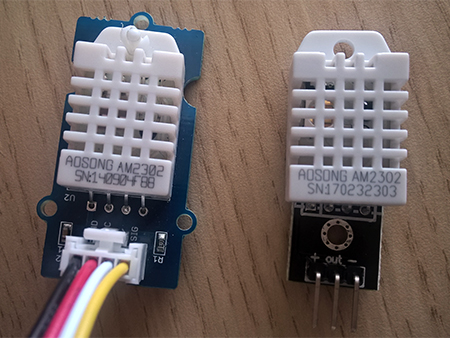• I saw your response in the other thread. I'll give it a try on the Lopy and let you know.